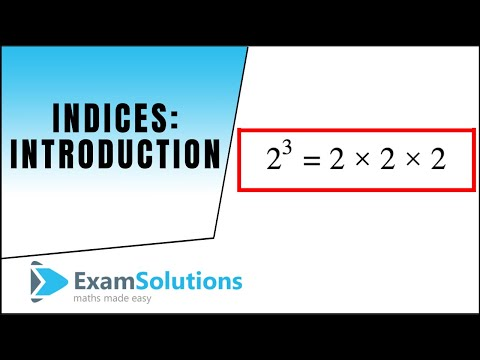## Indices

Subject: Compulsory Maths

Find Your Query

#### Overview

Indices are a useful way of more simply expressing large numbers. They also present us with many useful properties for manipulating them using what are called the Law of Indices.

##### Indices

Which is the greatest number in the following?(3)4, (9)3, (81)2

Here, the bases of all three terms and different. So it is difficult to compare. Therefore, converting all bases into the same base;

9 = 32

$\therefore$ 9= (32)= 32×3 = 36

Similarly, 81 = 34

$\therefore$ (81)= (34)= 34×2 = 38

Now, 3 is the base in 34, 36, 38. Therefore, the number having the greatest exponent with the same base is the greatest number.

∴ 38 or (81)2 is the greatest number.

Let us recall the laws of indices which we have studied in the previous classes.

1. Law of zero index: x0 = 1

2. Product law of indices: xm $\times$ xn = x m+n , (power are added in multiplication of same bases)

3. Power law of indices: (xm)n = x m$\times$h

4. Law of negative index: x-m = $\frac{1}{x^m}$

5. Root law of indices: x$\frac pq$ = $\sqrt[q]{x^p}$ = ($\sqrt[q]{x}$)p

6. Quotient law of indices: a÷ an = $\frac{a^m}{a^n}$ = $\frac{1}{a^{(n-m)}}$

($\frac{x}{y}$)n= $\frac{x^n}{y^n}$

7. (xy) m = xm ym

8. $\sqrt[n]{x}$ = x$\frac 1n$

These rules are known as laws of indices.

Example:

Find the value of: $\sqrt{8^2}$

$\sqrt{8^2}$

= 8$\frac{2}{3}$

= 23×$\frac{2}{3}$

= 22

= 4Ans

#### Exponential equation:

Exponential equation is an algebraic equation where unknown variables appears as an exponent of a base. We equate the power if the base on both sides of a equation is equal. We use the following rules while solving exponential equation:

1. If ax = ay then x = y

2. If ax = 1, then ax= a0

∴ x = 0

3. If ax = k1, then a = k$\frac 1x$

4. If ax = by, then a = b$\frac yx$

##### Things to remember

Some useful  formulas:

• x0 = 1
• xm $\times$ xn = x m+n , (power are added in multiplication of same bases)
• (xm)n = x m$\times$
• (xy) m = xm ym
• ($\frac{x}{y}$)= $\frac{x^n}{y^n}$
• x$\frac pq$ = $\sqrt[q]{x^p}$=($\sqrt[q]{x}$)p
• $\sqrt[n]{x}$ = x$\frac 1n$
• x-m = $\frac{1}{x^m}$
• It includes every relationship which established among the people.
• There can be more than one community in a society. Community smaller than society.
• It is a network of social relationships which cannot see or touched.
• common interests and common objectives are not necessary for society.
##### Videos for Indices##### Questions and Answers

Soln:$\sqrt{3}{8x^{-3}}$

=$\sqrt\frac{8}{x^3}$

= ($\frac {8}{x^3}$)$\frac 13$

={ ($\frac {2}{x}$)$^3$}$\frac 13$

= $\frac{2}{x}$ Ans.

Solu:${\left( \frac{5^{\frac{4}{3}}.5^{\frac{4}{3}}}{25^{\frac{1}{2}}} \right)}^\frac{3}{4}$

= ${\left( \frac{5^{\frac{4}{3}}.-^{\frac{1}{3}}}{5^2{\frac{1}{2}}} \right)}^\frac{3}{4}$

= ${\left( \frac{5^{\frac{4-1}{3}}}{5} \right)}^\frac{3}{4}$

= ${\left( \frac{5^{\frac{3}{3}}}{5} \right)}^\frac{3}{4}$

= ${\left( \frac{5^1}{5} \right)}^\frac{3}{4}$

= (1)$\frac{3}{4}$

Soln: ${\left( \frac{x^0}{64} \right)}^\frac{-2}{3}$

=${\left( \frac{1}{64} \right)}^\frac{-2}{3}$

=${\left( \frac{1}{4^3} \right)}^\frac{-2}{3}$

=${\left(4^{-3} \right)}^\frac{-2}{3}$

= (4)2

=16 Ans.

Soln:($\sqrt{3}$)6

=($\sqrt{3^\frac{1}{2}}$)6

= 33

= 27 Ans.

Soln: 4x=43

∴ x=3 Ans.

To verify: Put the value of x in given equation,

43=64 is true.

Soln:27x=3x+4

or, 33x=3x+4

or, 3x=x+4

or, 3x-x=4

or, 2x=4

∴ x=4/2= 2 Ans.

To verify: Put the value of x in given equation,

272=3x+4

or, 729= 36

or,729=729 is true.

Soln:92x=33-2x

or, (32)2x=33-2x

or, 34x=33-2x

or, 4x=3-2x

or, 4x+2x=3

or, 6x=3

∴ x=$\frac{3}{6}$=$\frac{1}{2}$ Ans.

Now, putting the value of x in given equation for the verification.

92×$\frac{1}{2}$=33-2×$\frac{1}{2}$

or, 9=33-1

or, 9=32

or, 9=9 is true.

Soln: 2×83=2x-4

or, 2×(22)3 =2x-4

or, 21×29=2x-4

or, 210=2x-4

or,10=x-4

or, 10+4=x

∴x=14 Ans.

To verify: Put the value of x in given equation.

2×83=214-4

or, 2× (23)3=210

or, 21×29=210

or,210=210 is true.

Soln:2x+1-2x-8=0

or,2x+1-2x=8

or, 2x.2-2x=8

or,2x(2-1)=23

or, 2x.1=23

∴x=3 Ans.

To verify: Put the value of x in given equation

23+1-23-8=0

or, 24-8-8=0

or, 16-16=0

or, 0=0 is true.

Soln:2x+3.3x+4=18

or,2x×23×3x×34 =18

or,2x×8×3x×81 =18

or,2x×3x=$\frac{18}{8×81}$

or, (2×3)x=$\frac{1}{36}$

or, 6x=$\frac{1}{6^2}$

or, 6x=6-2

∴x=-2 Ans.

To verify: Put the value of x given equation

2-2+3.3-2+4=18

or, 21.32=18

or, 2.9=18

or, 18=18 is true.

Soln:22x-3.5x-1=200

or,22x×2-3×5x×5-1=200

or,22x×5x=$\frac{200}{2^{-3}.5^{-1}}$

or,4x.5x =200× 23× 5

or,(4×5)x=200×8×5

or, (20)x=8000

or, (20)x=8000

or,(20)x=(20)3

∴x=3 Ans.

To verify: Put the value of x given equation

26-3.33-1=200

or, 23.52=200

or, 8 25=200

or, 200=200 is true.

Soln:2x-4=4x-6

or,2x-4=22(x-6)

or,2x-4=22x-12

or,x-4=2x-12

or,x-2x=-12+4

or, -x=-8

∴x=8 Ans.

To verify: Put the value of x given equation

28-4=48-6

or, 24=42

or,16=16 is true

Soln:2x+2=$\frac{2^{x+3}}{2}$=1

or, $\frac{2^{x+2.2^1+2^x+3}}{2}$=1

or,2x+2+1.2x+3=2

or,2x+3.2x+3=2

or,2x+3(1+1)=2

or, 2x+3.2=2

or, 2x+3=$\frac{2}{2}$

or,2x+3=1

or,2x+3=0

∴x=-3 Ans.

To verify: Put the value of x given equation

2x+2+$\frac{2^{x+3}}{2}$=1

or,2-3+2+$\frac{2^{-3+3}}{2}$=1

or,2-1+$\frac{2^0}{2}$=1

or,$\frac{1}{2}$$\frac{1}{2}$=1 [∴2-1=$\frac{1}{2}$ and 20=1]

or, $\frac{1+1}{2}$

or, $\frac{2}{2}$=1

or, 1=1 is true

Here, 3x=27

or, 3x=33

∴ x=3

##### Quiz

© 2019-20 Kullabs. All Rights Reserved.